Dimensions, Units, Conversion Factors, and Significant Digits

• Introduction
• There is a difference between dimensions and units. A dimension is a measure of a physical variable (without numerical values), while a unit is a way to assign a number or measurement to that dimension.
• For example, length is a dimension, but it is measured in units of feet (ft) or meters (m).
• There are three primary unit systems in use today:
• the International System of Units (SI units, from Le Systeme International d’Unites, more commonly simply called metric units)
• the English Engineering System of Units (commonly called English units)
• the British Gravitational System of Units (BG)
• The latter two are similar, except for the choice of primary mass unit and use of the degree symbol, as discussed below.
• Primary dimensions and units
• In total, there are seven primary dimensions. Primary (sometimes called basic) dimensions are defined as independent or fundamental dimensions, from which other dimensions can be obtained.
• The primary dimensions are: mass, length, time, temperature, electric current, amount of light, and amount of matter. For most mechanical and thermal science analyses, however, only the first four of these are required. The others will not be of concern to most mechanical engineering analyses.
• In order to assign numbers to these primary dimensions, primary units must be assigned. These are listed in the table below for the three unit systems:  Primary Dimension Symbol SI unit BG unit English unit mass m (sometimes M) kg (kilogram) slug lbm (pound-mass) length L (sometimes l) m (meter) ft (foot) ft (foot) time t (sometimes T) s (second) s (second) s (second) temperature T (sometimes q ) K (Kelvin) oR (degree Rankine) R (Rankine) electric current I (sometimes i) A (ampere) A (ampere) A (ampere) amount of light (luminous intensity) C (sometimes I) c (candela) c (candela) c (candela) amount of matter n or N (sometimes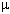) mol (mole) mol (mole) mol (mole)
• All other dimensions can be derived as combinations of these seven primary dimensions. These are called secondary dimensions, with their corresponding secondary units. A few examples are given in the table below:  Secondary Dimension Symbol SI unit BG unit English unit force F (sometimes f) N (Newton = kg× m/s2) lbf (pound-force) lbf (pound-force) acceleration a m/s2 ft/s2 ft/s2 pressure p or P N/m2, i.e. Pa (Pascal) lbf/ft2 (psf) lbf/in2 (psi) (note: 1 ft = 12 in) energy E (sometimes e) J (Joule = N× m) ft× lbf (foot pound) ft× lbf (foot pound) power P W (watt = J/s) ft× lbf/s ft× lbf/s
• Note that there are many other units, both metric and English, in use today. For example, power is often expressed in units of Btu/hr, Btu/s, cal/s, ergs/s, or horsepower, in addition to the standard units of watt and ft× lbf/s. There are conversion factors listed in many textbooks to enable conversion from any of these units to any other.
• Comment about the gravitational conversion constant, gc
• Some authors define a gravitational conversion constant, gc, which is inserted into Newton’s second law of motion. I.e., instead of F = m× a, they write F = m× a/gc, where gc is defined in the English Engineering System of Units asand in SI units as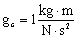• The present author discourages use of this constant, since it leads to much confusion. Instead, Newton’s law should remain in the fundamental form in which it was created, without an artificial constant thrown into the equation, simply for unit’s sake.
• There has been much confusion (and numerical error!) because of the differences between lbf, lbm, and slug. The use of gc has complicated and further confused the issue, in this author’s opinion. The following is an attempt to clarify some of this confusion:
• The relationship between force and mass units
• The relationship between force, mass, and acceleration can be clearly understood with Newton’s second law. The following is provided to avoid confusion, especially with English units.
• SI units:  Relationship Newton’s second law, F = m a. [Note: Bold notation indicates a vector.] By definition of the fundamental units, this yields 1 N = 1 kg× m/s2. Conversion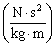Discussion The above expression is dimensionless and has a value of 1. Thus it is the conversion factor with which to multiply or divide any equation to simplify the units. Example How much force (in Newtons) is required to accelerate a mass of 13.3 kg at a constant acceleration of 1.20 m/s2?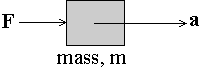Solution: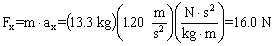to the right, since Fx is the x-component of vector F, and ax is the x-component of acceleration vector a. Terminology It is not proper to say that 1.00 kg equals 9.81 N, but it is proper to say that 1.00 kg weighs 9.81 N under standard earth gravity. This is obtained by utilizing Newton’s second law with gravitational acceleration, i.e.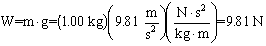.
• English units:  Relationship Newton’s second law, F = m a. [Note: Bold notation indicates a vector.] By definition of the fundamental units, this yields 1 lbf = 1 slug× ft/s2, or 1 lbf = 32.174 lbm× ft/s2. Conversion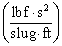ororDiscussion The above expressions are dimensionless and each has a value of 1. Thus any of them can be considered a conversion factor with which to multiply or divide any equation to simplify the units. Example How much force (in lbf) is required to accelerate a mass of 13.3 lbm at a constant acceleration of 1.20 ft/s2?Solution: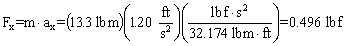to the right, since Fx is the x-component of vector F, and ax is the x-component of acceleration vector a. Terminology It is not proper to say that one lbm equals one lbf, but it is proper to say that one lbm weighs one lbf under standard earth gravity. This is obtained by utilizing Newton’s second law with gravitational acceleration, i.e.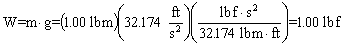.
• The Principle of Dimensional Homogeneity
In any equation, each additive term must have the same dimensions. In simple terms, you cannot add apples and oranges.
• Example - The area of a rectangle is the product of its width and its height, A = W H. The dimensions of both terms in this equation are {length2}. The equation A = H is clearly wrong, i.e. it is dimensionally inconsistent since the dimensions of the left term are {length2} while those of the right term are {length}.
• The Principle of Dimensional Homogeneity is sometimes useful when checking the algebra of a problem solution. Namely, dimensional inconsistency in an equation is a sure sign of an algebraic error!
• The Principle of Dimensional Homogeneity also extends to units. The best way to avoid unit errors is to list the units along with any numbers supplied to an equation. Also, it is best to introduce conversion factors in the form of ratios. In the above example, suppose the width W of the rectangle is 48.0 inches, and the Height H is 2.0 feet. The area A is desired in square feet, and is calculated correctly as follows:
A = W H = (48.0 in) (2.0 ft) (1 ft / 12 in) = 8.0 ft2.

• Significant Digits
Since the proliferation of calculators in the 1970's, the concept of significant digits has been largely ignored. As a result, many students and practicing engineers today present answers to five, six, or more digits, even when only two or three digits are significant. Many students, for example, will write out every digit (perhaps eight or ten) that is displayed on their calculators, never even thinking about how many of those digits are actually meaningful. The present author encourages all students and engineers to consider significant digits in all written forms of communication - reports, papers, homework, exams, etc. Below is a discussion of the meaning and application of significant digits in engineering.
• By default, an integer has an infinite number of significant digits. For example, the number 43 implies exactly 43, as when counting the number of students in a classroom. Unfortunately, many authors do not follow this convention, and it is unclear to the reader how many significant digits there really are, especially when there are trailing zeroes.
• The number of significant digits is determined by the overall accuracy of a measurement. For example, suppose the diameter of a pipe is measured to be 2.53 mm. By convention, the measurement is only good to the least significant digit; here the micrometer is accurate to 0.01 mm, but the exact diameter may lie anywhere between 2.525 and 2.535 mm. In this example, the reading is good to three significant digits.
• When considering the number of significant digits, leading zeroes for numbers below unity do not count, but zeroes within a value do count. For example, 0.367 has three significant digits - the leading zero does not count. Note that this same value can be written in exponential notation as 3.67 x 10-1, where the number of significant digits is more obvious. Consider the value 34.05. The zero here does count, so that the value has four significant digits.
• Trailing zeroes are a little more tricky, especially when not using exponential notation. For example, suppose a pressure reading of 101,300 Pascals is given. It is not obvious how many (if any) of the trailing zeroes are significant. Most likely, the pressure gage is only accurate to a hundred Pascals, so it is more appropriate to write this measurement as 101.3 kPa, avoiding the trailing zeroes altogether. The number of significant digits in this case is four. A reading of 101.30 kPa implies that the trailing zero is significant, and the total number of significant digits is five.
• If trailing zeroes are significant, there are two ways to indicate this: First, use exponential notation, which clearly indicates the accuracy. For example, if a reading of 1000 is accurate to all four digits, one would write it as 1.000 x 103. Second, one can write "1000." as the numerical value. The decimal point at the end of the number indicates that all three zeroes are significant. It is understood, then, that "1000." represents four significant digits of accuracy. In this same example, if only three digits are significant, one would write the value as 1.00 x 103. If exponential notation is not desired, but one still wishes to indicate the number of digits, one can write "1000 to three significant digits".
• Here is an important rule to remember: When performing calculations or manipulations of several parameters, the final result is only as accurate as the least accurate parameter in the problem. For example, suppose A and B are multiplied to obtain C. If A = 2.3601 (five significant digits), and B = 0.34 (two significant digits), then C = 0.80 (only two digits are significant in the final result). Note that most students are tempted to write C = 0.802434, with six significant digits, since that is what is displayed on a calculator after multiplying these two numbers. Let's analyze this simple example carefully. Suppose the exact value of B is 0.33501, which is read by the instrument as 0.34. Also suppose A is exactly 2.3601, as measured by a more accurate instrument. In this case, C = A times B = 0.79066 to five significant digits. Note that our first answer, C = 0.80 is off by one digit in the second decimal place. Likewise, if B is 0.34499, read by the instrument as 0.34, the product of A and B would be 0.81421 to five significant digits. Our original answer of 0.80 is again off by one digit in the second decimal place. The main point here is that 0.80 (to two significant digits) is the best we can expect from this multiplication since, to begin with, one of the values had only two significant digits. Another way of looking at this is to say that beyond the first two digits in the answer, the rest of the digits are meaningless or not significant. For example, if one reports what his calculator displays, i.e. 2.3601 times 0.34 equals 0.802434, the last four digits are meaningless. As shown above, the final result may lie between 0.79 and 0.81 - any digits beyond the two significant digits are not only meaninless, but misleading, since it implies more accuracy to the reader than is really there.
• Most electronic instruments are good to only three significant digits. When in doubt, for most engineering analyses, three digits are usually the maximum that can be expected.
• When writing out intermediate results in a calculation, it is okay to record more digits than the number which is significant, as this can avoid round-off errors in subsequent calculations. However, when displaying the final answer, the number of significant digits should be taken into consideration.